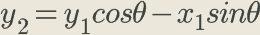# Introduction

This post is a continuation of the previous one, so it would be wise to read that first if you haven’t already. The question I seek to answer here is similar to the last one: Will a system behave the same if oriented differently? I’ve already shown that systems are symmetric translationally, so now I’ll show that mechanics is also rotationally symmetric. That is to say identical (excluding orientation) systems behave identically regardless of orientation.

# The Proof

I shall once again use the equation for force in this proof. This exercise is a bit more complex than the last because we must include both the x and y dimensions, whereas previously only the x dimension was required. This will also require a basic understanding of trigonometry, as angles will be involved. We begin by defining the coordinates. The origin (before the rotation) is defined by the coordinates x1 and y1. The rotated coordinates, then, are x2 and y2, which are rotated by some angle θ. These can be expressed in terms of x1 and y1 like this:Where θ defines the rotation and can be used with the origin to calculate the rotated coordinates.

Where θ defines the rotation and can be used with the origin to calculate the rotated coordinates.

Now, since I’m using force for this proof, it will be helpful to define the relationship between the forces at each point.

Assuming physics functions correctly at the origin, the equations there are as follows:

Thus, the equations used to calculate force at the rotated coordinates are as follows:

If we can prove that the force calculated at the rotated point is equal to the force at the origin, then we can say that there is rotational symmetry. To do this, we can begin by defining the left side of the equations above in terms of the origin. I’ve already defined the relationship between the forces, so all that’s left to be done is to express force as the product of mass and acceleration.

Now we can work on the right side of those equations. I’ve already defined the relationship between the origin and the rotated coordinates to be

Assuming the angle θ to be constant, differentiating twice with respect to time yields the following:

Finally, we multiply every term by mass.

We now discover that the force calculated at (x1, y1) is exactly equal to the force calculated at (x2, y2). The same process can be repeated across the z-axis to incorporate all three physical dimensions. This proves that physics (mechanically) is rotationally symmetrical.

# Discussion and Conclusion

There you have it. Mechanics does indeed work identically across both translational and rotational transformations. This symmetry project was pretty enjoyable, as it allowed me to introduce some calculus into my posts. The physics class I’m currently taking is algebra-based, so it’s nice to flex the ol’ calculus muscles every once in a while. I’m working on a few different post ideas currently, all of which you’ll undoubtedly want to see. By the way, if you want to be notified every time I upload a new post, enter your email into the big green bar on the homepage. I post every few weeks or so, so your inbox won’t be assailed with emails.

P.S. Shout out to the homie Richard Feynman for the idea for this project.

--

--